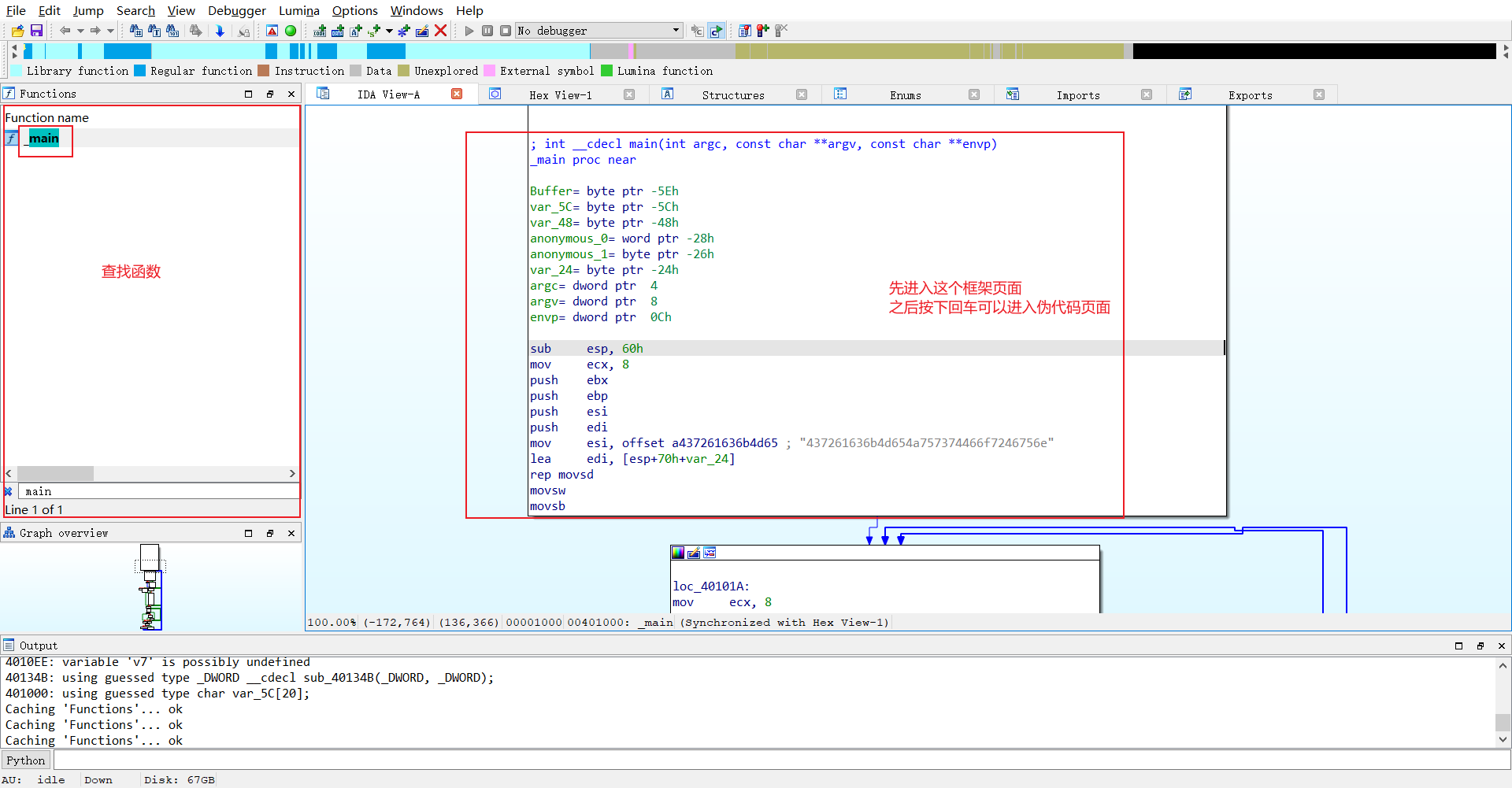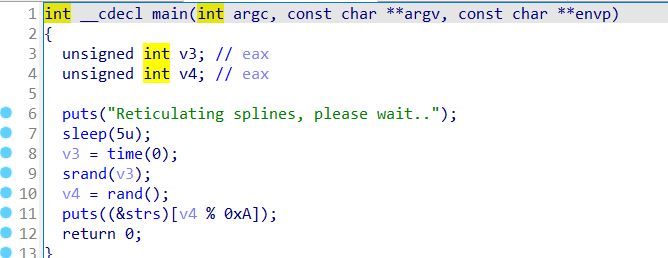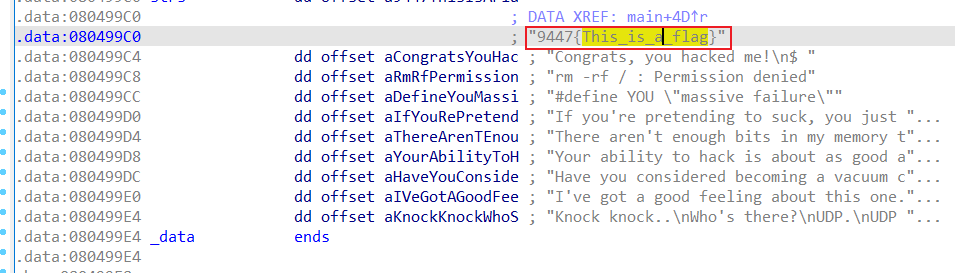# Reverse(一)

exe文件拖入后等待完全分析完后，在左边部分CTRL+F查找相关函数，入门应该都是查找main函数，双击进入后按下tab可以查看文件的伪代码## 1. Hello, CTF16进制转化下ASCII即可得到：CrackMeJustForFun

## 2. insanityimport base64

def encode(message):
s = ''
for i in message:
x = ord(i) ^ 32
x = x + 16
s += chr(x)

return base64.b64encode(s)

correct = 'XlNkVmtUI1MgXWBZXCFeKY+AaXNt'
flag = ''
print 'Input flag:'
flag = raw_input()
if encode(flag) == correct:
print 'correct'
else:
print 'wrong'

import base64

def decode(message):
s = ''
for i in message:
s += chr((i - 16) ^ 32)
return s

correct = 'XlNkVmtUI1MgXWBZXCFeKY+AaXNt'
flag = base64.b64decode(correct)
print(decode(flag))